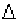Custom Search
If you want to discuss more on this or other issues related to Physics, feel free to leave a message on my Facebook page.

## Monday, October 5, 2009

### How to determine uncertainty in a derived quantity when addition or subtraction is performed?

As we have seen in the previous post it is important to know how to determine the uncertainty for derived quantities.

Let us see how to determine how to determine the uncertainty of a derived quantity when an addition or a subtraction is performed.

In an experiment two quantities are measured as shown.

A = 56.4 +_0.1 cm

B = 12.2+_0.1 cm

If a derived quantity C is given by the equation below

C = A + B

= o.2 cm

C = 56.4 + 12.2 = 68.6 cm

C = 68.6 +-0.2 cm

If you want to calculate C and the equation relating C to A and B is

C = A – B

Then we can use the following equation to calculateC

If C = 56.4 – 12.2 = 44.2 cm

then C = 44.2 +-0.2 cm

This is then end of this part. Please follow the link below to go to the part on determining the